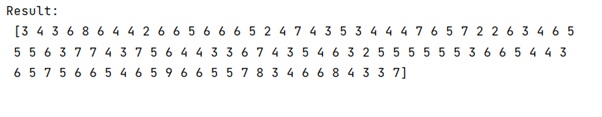# numpy.random.binomial() Method with Example

Python | numpy.polyfit(): Learn about the numpy.polyfit() method, its usages and example.
Submitted by Pranit Sharma, on February 27, 2023

NumPy is an abbreviated form of Numerical Python. It is used for different types of scientific operations in python. Numpy is a vast library in python which is used for almost every kind of scientific or mathematical operation. It is itself an array which is a collection of various methods and functions for processing the arrays.

## numpy.random.binomial() Method

This function is used to draw samples from a binomial distribution. In mathematics, the binomial distribution with parameters n and p is the discrete probability distribution in a sequence of n independent experiments which gives only two possible results either success or failure.

Samples are drawn from a binomial distribution with specified parameters, n trials, and p probability of success where n is an integer >= 0 and p is in the interval [0,1].

Syntax:

```random.binomial(n, p, size=None)
```

Parameter(s):

• n: parameters of the distribution which are greater than or equal to 0.
• p: parameters of the distribution which are greater than or equal to 0 and less than or equal to 1.
• size: shape of the output.

Let us understand with the help of an example,

## Python code to demonstrate the example of numpy.random.binomial() method

```# Import numpy
import numpy as np

# Defining number of trial
n = 10

# Define possibility
p = 0.5

# Draw binomial distribution
res = np.random.binomial(n,p,100)

# Display result
print("Result:\n",res,"\n")
```

Output:Languages: » C » C++ » C++ STL » Java » Data Structure » C#.Net » Android » Kotlin » SQL
Web Technologies: » PHP » Python » JavaScript » CSS » Ajax » Node.js » Web programming/HTML
Solved programs: » C » C++ » DS » Java » C#
Aptitude que. & ans.: » C » C++ » Java » DBMS
Interview que. & ans.: » C » Embedded C » Java » SEO » HR
CS Subjects: » CS Basics » O.S. » Networks » DBMS » Embedded Systems » Cloud Computing
» Machine learning » CS Organizations » Linux » DOS
More: » Articles » Puzzles » News/Updates